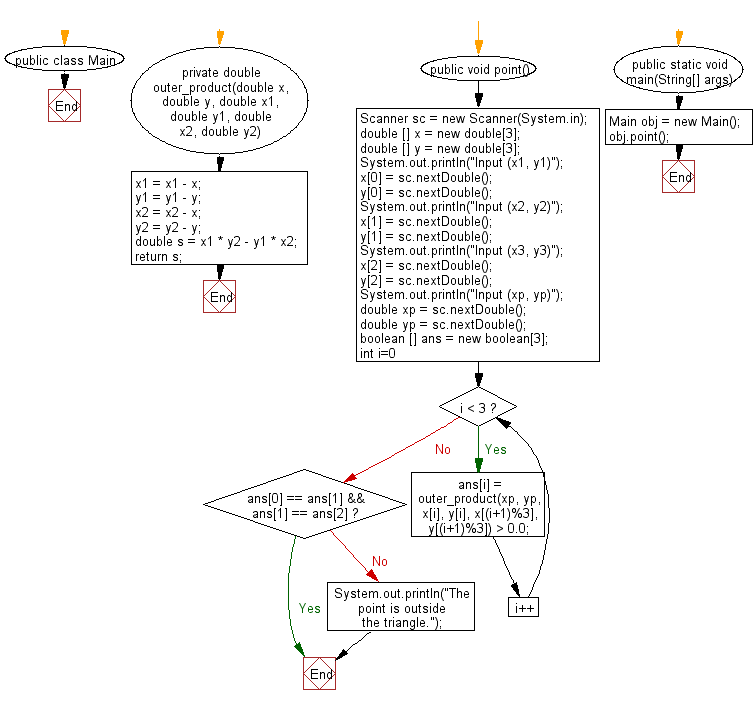# Java Exercises: Check if a point (x, y) is in a triangle or not

## Java Basic: Exercise-219 with Solution

Write a Java program to check if a point (x, y) is in a triangle or not. There is a triangle formed by three points.

Input:
x1,y1,x2,y2,x3,y3,xp,yp separated by a single space.

Sample Solution:

Java Code:

``````import java.util.*;
public class Main {
private double outer_product(double x, double y, double x1, double y1, double x2, double y2){
x1 = x1 - x;
y1 = y1 - y;
x2 = x2 - x;
y2 = y2 - y;
double s = x1 * y2 - y1 * x2;
return s;
}
public void point(){
Scanner sc = new Scanner(System.in);
double [] x = new double;
double [] y = new double;
System.out.println("Input (x1, y1)");
x = sc.nextDouble();
y = sc.nextDouble();
System.out.println("Input (x2, y2)");
x = sc.nextDouble();
y = sc.nextDouble();
System.out.println("Input (x3, y3)");
x = sc.nextDouble();
y = sc.nextDouble();
System.out.println("Input (xp, yp)");

double xp = sc.nextDouble();
double yp = sc.nextDouble();
boolean [] ans = new boolean;
for(int i=0; i < 3; i++){
ans[i] = outer_product(xp, yp, x[i], y[i], x[(i+1)%3], y[(i+1)%3]) > 0.0;
}
if(ans == ans && ans == ans){

}
else{
System.out.println("The point is outside the triangle.");
}
}
public static void main(String[] args) {
Main obj = new Main();
obj.point();
}
}

```
```

Sample Output:

```Input (x1, y1)
2
6
Input (x2, y2)
3
5
Input (x3, y3)
4
6
Input (xp, yp)
5
6
The point is outside the triangle.
```

Flowchart:Java Code Editor:

What is the difficulty level of this exercise?

﻿

## Java: Tips of the Day

Parsing dates:

```import java.io.*;
import java.util.*;
import java.text.*;

String s = "2001/09/23 14:39";

SimpleDateFormat formatter = new SimpleDateFormat ("yyyy/MM/dd H:mm");
Date d = formatter.parse(s, new ParsePosition(0));
```